﻿ Graphing functions like y = a sin(k(x-b)) and y = a cos(kx+b)

# GRAPHING GENERALIZED SINES AND COSINES, LIKE $\,y = a\sin k(x\pm b)\,$ and $\,y = a\cos (kx\pm B)\,$

LESSON READ-THROUGH (Part 1 of 2)
by Dr. Carol JVF Burns (website creator)
Follow along with the highlighted text while you listen!
Thanks for your support!
• PRACTICE (online exercises and printable worksheets)

The prior section explored the (basic) graphs of $\,y = \sin x\,$ and $\,y = \cos x\,$.
However, these trigonometric functions frequently appear in ‘transformed’ versions.
You might see, for example, $\,y = -1.5\sin \frac{1}{2}(x - \pi)\,$ or $\,y = \frac{1}{3}\cos (4x + 5)\,$.

The observations and techniques discussed in this section will allow you to reliably, quickly, and efficiently graph any function of the following forms, without memorizing a bunch of different formulas:

 the ‘generalized functions’ addressed in this section: $y = a\sin k(x\pm b)$ $y = a\cos k(x\pm b)$ $y = a\sin (kx\pm B)$ $y = a\cos (kx\pm B)$

Notice, in particular, that the arguments $\,k(x\pm b)\,$ (first row) and $\,kx\pm B\,$ (second row) are different.
For example, $\,2(x-5)\,$ is different from $\,2x - 5\,$.
These input types are commonly seen in applications.

In mathematics, the word ‘argument’ is often used to refer to the input of a function.
For example, the argument of the sine function in the expression ‘$\,\sin k(x\pm b)\,$’ is $\,k(x\pm b)\,$.
As a second example, the argument of the cosine function in the expression ‘$\,\cos (kx\pm B)\,$’ is $\,kx\pm B\,$.

Any argument with only two types of terms—an $\,x\,$-term and a constant term—
can be handled with the four-step graphing process discussed below.

With this four-step technique, you don't need to identify which type of argument you're working with;
you only need to identify the coefficient of the $\,x\,$-term.

In this section,
the coefficient of the $\,x\,$-term in the argument is always denoted by $\,k\,$.

For example, the four-step technique can be used to graph (say) $\displaystyle\,y = -9\sin\frac{\pi + 5x}{8}\,$.
Why? The argument has only an $x$ term and a constant term.

Here, $\,k = \frac 58\,$.
Why? Rewrite the argument (as needed) to see that the coefficient of $\,x\,$ is $\,\color{red}{\frac 58}\,$: $$\cssId{s27}{\frac{\pi +\color{red}{5}x}{\color{red}{8}} = \frac{\pi}8 + \color{red}{\frac{5}{8}}x}\,$$

The following two key observations will lead to an efficient approach.
Click the show/hide buttons for additional information.

1. Observation #1:
Each generalized function is the result of graphical transformation(s) chosen from:
2. Observation #2:
The only transformation that changes the period is the horizontal stretch/shrink.

The horizontal stretch/shrink is caused by replacing every $\,x\,$ by $\,kx\,$,
which causes points $\,(x,y)\,$ to move to new points $\displaystyle\,(\frac{x}{k},y)\,$.

For $\,k > 0\,$, the horizontal stretch/shrink changes the original period $\,2\pi\,$ to the new period $\displaystyle\,\frac{2\pi}{k}\,$.

If $\,k < 0\,$, rewrite first, using (as needed):
This leads us to:

## THE FOUR-STEP GRAPHING PROCESS:

You'll graph only one period.
By extending periodically, this uniquely defines the entire curve.

GENERAL PROCEDURE EXAMPLE:   Graph $\,y = -7\cos (3-5x)\,$
STEP 1:   CHECK THAT $\,k\,$ IS POSITIVE

The coefficient of the $\,x\,$-term in the argument is denoted by $\,k\,$.
Make sure that $\,k > 0\,$.
If not, rewrite (as above), using the odd/even properties of sine/cosine.
Initially, the coefficient of the $\,x\,$ term is $\,-5\,$.

Rewrite:
$$\, \cssId{s62}{-7\cos (3-5x)}\ \ \cssId{s63}{\underset{\text{law}}{\overset{\text{distributive}}{=}}}\ \ \cssId{s64}{-7\cos\bigl(-(5x-3)\bigr)}\ \ \cssId{s65}{\underset{\text{is even}}{\overset{\text{cosine}}{=}}}\ \ \cssId{s66}{-7\cos (5x-3)}$$
Instead, graph:   $y = -7\cos (5x-3)$
(So, $\,k = 5\,$.)
STEP 2:   STARTING POINT

Each sine/cosine curve starts a ‘natural’ cycle when its argument is zero.
Compute the value of $\,x\,$ that makes the argument equal to zero.
This is your starting point; call it $\,S\,$ and mark it on a number line.
Find $\,S\,$: $$\begin{gather} \cssId{s74}{5x-3 = 0}\cr \cssId{s75}{5x = 3}\cr \cssId{s76}{x = \frac{3}{5}} \end{gather}$$ So, $\,S = \frac{3}{5}\,$.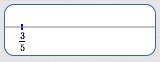STEP 3:   ENDING POINT

Compute the new period, $\displaystyle\,\frac{2\pi}{k}\,$.

The cycle that starts at $\,S\,$ ends at $\displaystyle\,E := S +\frac{2\pi}{k}\,$.
In other words, one complete cycle occurs on the interval $\,[S,\underbrace{S +\frac{2\pi}{k}}_{E}]\,$.

Mark the ending point, $\,E\,$, on the number line.

Divide the interval from STARTING POINT to ENDING POINT into four equal parts, to make it easier to draw in the basic cycle.
$\,k = 5\,$

$\cssId{s85}{\displaystyle\text{period} = \frac{2\pi}{k} = \frac{2\pi}{5}}$

$\cssId{s86}E := S + \frac{2\pi}{k} = \frac{3}{5} + \frac{2\pi}{5}$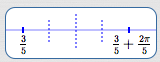STEP 4:   DRAW IN THE BASIC CYCLE;
ADJUST (AS NEEDED) FOR AMPLITUDE/FLIP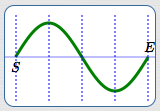basic sine cycle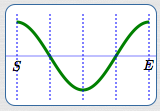basic cosine cycle

As discussed in the next section, amplitude is the distance from the $x$-axis to the highest (or lowest) part of the graph.

The amplitude of $\,y = \sin x\,$ and $\,y = \cos x\,$ is $\,1\,$.
Vertical scaling by a factor of $\,a\,$ causes the amplitude to change to $\,|a|\,$.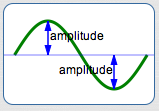Vertical scaling by a factor of $\,-1\,$ causes reflection about
the $x$-axis (i.e., a ‘flip’).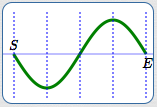basic sine cycle, flipped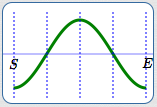basic cosine cycle, flipped
Draw in the basic cosine cycle, flipped.

The amplitude is $\,7\,$.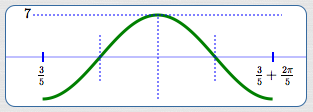To emphasize:
In this graph of (one cycle of) $y = -7\cos (5x-3)$:
• the fact that it is a cosine curve, $\,y = -7\color{red}{\cos}(5x-3)\,$, causes you to use the basic cosine cycle;
• the leading minus sign, $\,{\bf\color{red}{-}}7\,$, causes the cycle to be flipped;
• the amplitude is $\,|-7|\, = 7\,$

## EXAMPLES

The three earlier examples are repeated, using a compact version of the four-step graphing process.
A new example is also given.
See how quick and easy it is!

In these examples, cycles are shown in a standard viewing window ($\,x\,$ between $\,-20\,$ and $\,20\,$; $\,y\,$ between $\,-10\,$ and $\,10\,$),
to make comparisons between graphs easier.
The cycle being graphed with the four-step process is shown in dark green.
Additional cycles are shown to complete the viewing window.
Decimal approximations to the tenths place are included.
Remember to get exact answers first, then approximate!

### Graph $\,y = -1.5\sin\frac12(x-\pi)\,$ using the four-step process

1. $k = \frac 12 = 0.5$
2. $S = \pi \approx 3.1$
(mentally set $\,\frac 12(x-\pi)\,$ equal to zero)
3. $\displaystyle\text{period} = \frac{2\pi}{k} = \frac{2\pi}{0.5} = 4\pi \approx 12.6$
$E = S + \text{period} = \pi + 4\pi = 5\pi \approx 15.7$
4. basic sine cycle, flipped, on about $\,[3.1,15.7]\,$, amplitude is $\,1.5\,$: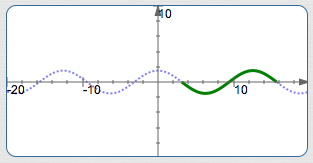### Graph $\,y = \frac13\cos (4x+5)\,$ using the four-step process

1. $k = 4$
2. $S = -\frac{5}{4} = -1.25$
(mentally set $\,4x+5\,$ equal to zero)
3. $\displaystyle\text{period} = \frac{2\pi}{k} = \frac{2\pi}{4} = \frac{\pi}{2} \approx 1.6$
$\displaystyle\displaystyle E = S + \text{period} = -1.25 + \frac{\pi}2 \approx 0.3$
4. basic cosine cycle on (about) $\,[-1.25,0.3]\,$, amplitude is $\displaystyle\,\frac{1}{3}\,$: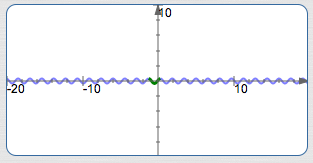### Graph $\,y = -7\cos (3 - 5x)\,$ using the four-step process

1. Rewrite: $-7\cos(3-5x) = -7\cos(-(5x-3)) = -7\cos(5x-3)$
(cosine is even)
$k = 5$
2. $S = \frac{3}{5} = 0.6$
(mentally set $\,5x-3\,$ equal to zero)
3. $\displaystyle\text{period} = \frac{2\pi}{5} = 0.4\pi \approx 1.3$
$E = S + \text{period} = 0.6 + 0.4\pi \approx 1.9$
4. basic cosine cycle, flipped, on about $\,[0.6,1.9]\,$, amplitude is $\,7\,$: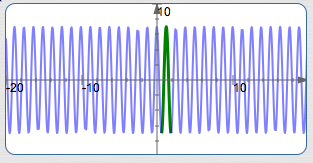### Graph $\displaystyle\,y = -4\sin\frac{\pi-3x}{8} \,$ using the four-step process

1. Rewrite: $\displaystyle -4\sin\frac{\pi-3x}{8} = -4\sin (-\frac 18(3x-\pi)) = 4\sin\frac18(3x - \pi)$
(sine is odd)
$\displaystyle k = \frac38 \approx 0.4$
2. $\displaystyle S = \frac{\pi}{3} \approx 1.0$
(mentally set $\displaystyle\,\frac18(3x-\pi)\,$ equal to zero)
3. $\displaystyle\text{period} = \frac{2\pi}{3/8} = \frac{16\pi}3 \approx 16.8$
$\displaystyle E = S + \text{period} = \frac{\pi}3 + \frac{16\pi}3 = \frac{17\pi}3 \approx 17.8$
4. basic sine cycle on about $\,[1.0,17.8]\,$, amplitude is $\,4\,$: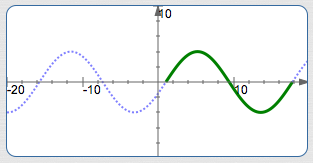Master the ideas from this section
by practicing the exercise at the bottom of this page.

When you're done practicing, move on to:
Amplitude, Period, and Phase Shift
On this exercise, you will not key in your answer.
However, you can check to see if your answer is correct.
PROBLEM TYPES:
 1 2 3 4 5 6 7 8 9 10 11 12 13 14 15 16 17 18 19
AVAILABLE MASTERED IN PROGRESS
 (MAX is 19; there are 19 different problem types.) Want textboxes to type in your answers? Check here: